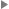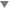## superscript

superscript — A superscript (as in x^2, the mathematical notation for x multiplied by itself).

## Description

A `superscript` identifies text that is to be displayed as a superscript when rendered.

### Processing expectations

Formatted inline. Superscripts are usually printed in a smaller font and shifted up with respect to the baseline.

#### ChildrenThis element contains 16 elements.

## Examples

```  1 <article xmlns='http://docbook.org/ns/docbook'>
2 <title>Example superscript</title>

4 <para>The equation e<superscript>πi</superscript> + 1 = 0 ties together
five of the most important mathematical constants.
6 </para>

8 </article>```

The equation eπi + 1 = 0 ties together five of the most important mathematical constants.

Last revised by Norman Walsh on 6 Jun 2011 (git hash: 6ffcc7640bbc5f852a318e452c9f210f03292cb9)